Imagination is more important than knowledge... Albert Einstein

Guess is more important than calculation --- Knowhowacademy.com

Number - Simple Calculation

For SAT1
Number of Total Problems: 40.
FOR PRINT ::: (Book)

 Problem Num : 1 Type: None Section:Number Theme: Adjustment# : Difficulty: 1Category Simple Calculation
Analysis

 Problem Num : 2 Type: None Section:Number Theme: Adjustment# : Difficulty: 1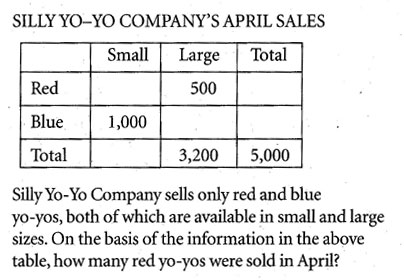Category Simple Calculation
Analysis

 Problem Num : 3 Type: Calculation Section:Number Theme: Adjustment# : 0 Difficulty: 1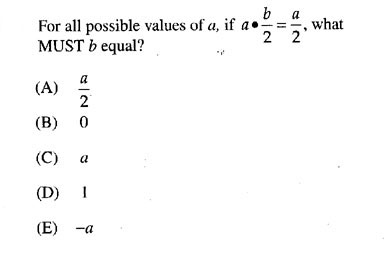Category Simple Calculation
Analysis

 Problem Num : 4 Type: None Section:Number Theme: Adjustment# : 0 Difficulty: 1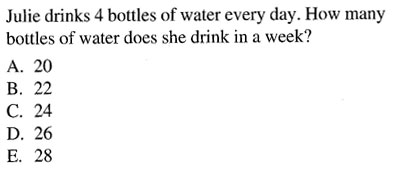Category Simple Calculation
Analysis

 Problem Num : 5 Type: None Section:Number Theme: Adjustment# : 0 Difficulty: 1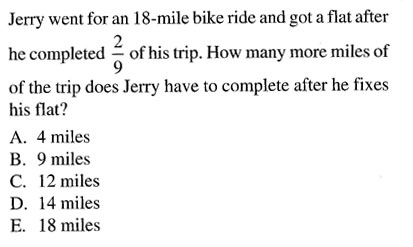Category Simple Calculation
Analysis

 Problem Num : 6 Type: None Section:Number Theme: Adjustment# : 0 Difficulty: 1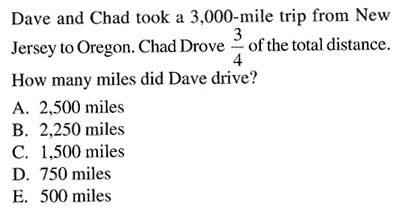Category Simple Calculation
Analysis

 Problem Num : 7 Type: Understanding Section:Number Theme: Adjustment# : 0 Difficulty: 1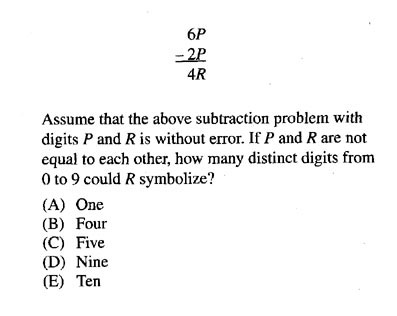Category Simple Calculation
Analysis

 Problem Num : 8 Type: Calculation Section:Number Theme: Adjustment# : 0 Difficulty: 1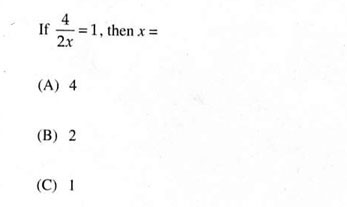Category Simple Calculation
Analysis

 Problem Num : 9 Type: Calculation Section:Number Theme: Adjustment# : 0 Difficulty: 1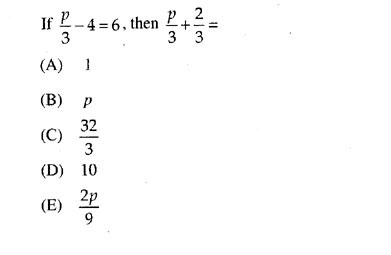Category Simple Calculation
Analysis

 Problem Num : 10 Type: Word problem Section:Number Theme: Adjustment# : 0 Difficulty: 1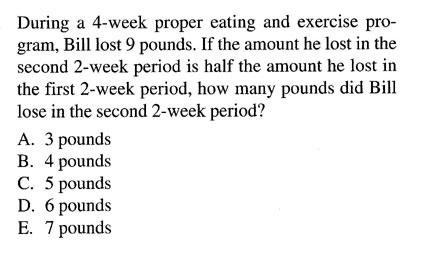Category Simple Calculation
Analysis